Publicité

# CE 8394 FLUID MECHANICS AND MACHINERY

18 May 2019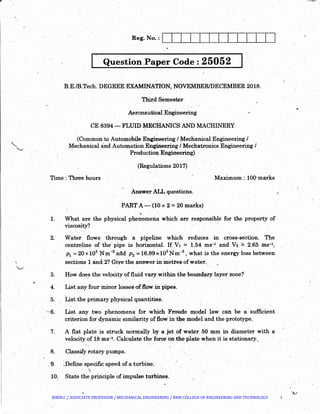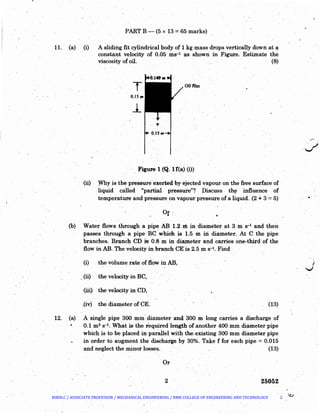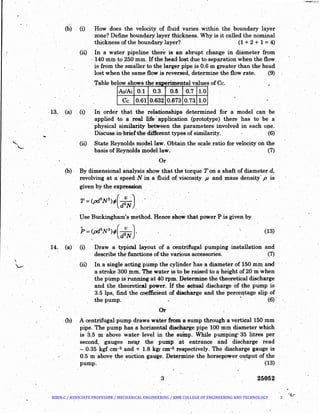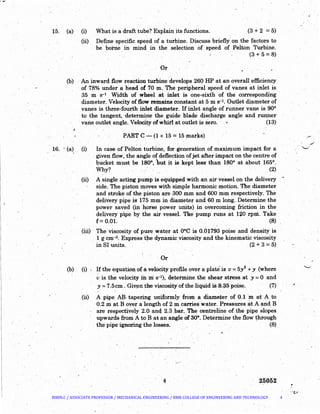Prochain SlideShare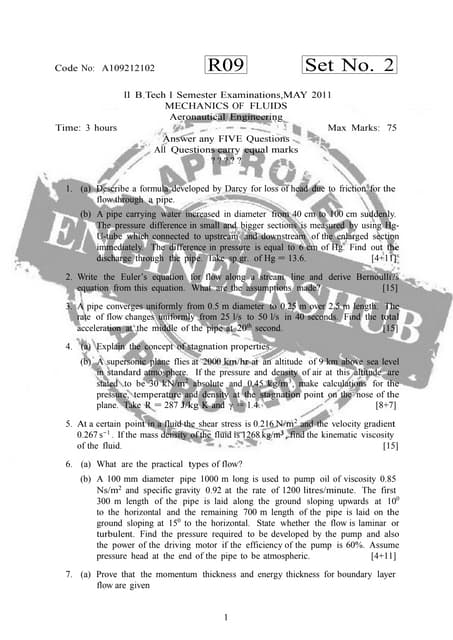A109212102 mechanicsoffluids1
Chargement dans ... 3
1 sur 4
Publicité

### CE 8394 FLUID MECHANICS AND MACHINERY

1. Reg. No. :l....___._I_.· _._,___.___.__,__..._..__-.-'-'-1_.._____.__~ ) ' Question_Paper Code: 25052 · (-· B.E.lB.Tech. DEGREE EXAMINATION, NOVEMBERIDECE;MBER 2018. Third Semester Aeronautical Engineering .•' CE 8394 -. FLUID MECHANICS AND MACHINERY (Common to Automobile Engineering/ Mechanical Engineering I Mechanical and Automation Engineering I Mechatronics Engineering I · Production Engineering) (Regulations 2017) Time : Three hours Maximum : 100 marks 1. 2. 3. AnswerALL questions. PART A-·.(10 x2 ~ 20 marks) "What are the physica(ph.enomep.a which are responsible for the. property of viscosity? Water flows through a pipeline which reduces in cross~section. The centreline of the pipe is horizontal. If v1 =1,54 ms-1and v~ =. 2.65 ms-1, p1 =20 x 103 N m'"2 and p 2 =16..89 x103 N m-2 , what is the energy loss between sections 1 and 2? Giye the answer in metres of water; How doe_s the velocity of fluid vary_within the boundary layer zone? 4. List any four minor losses-offlow in pipes. 5. List the primary physical quantities. -C~6. List any two phenomena. for which Froude model law can be a sufficient criterion for dynamic similarity of.tlow in ~e modeland the prototype.. 7. A flat plate is struck normally by a jet of water 50 mm in diameter with a velocity of 18 ms-1• C~llculate the force on the plate when it is stationary.. 8. Classify rotary pumps. . 9. ~Define specific speed of a turbine. . ' 10. State the principle of impulse tu,rhines. · ... BIBIN.C / ASSOCIATE PROFESSOR / MECHANICAL ENGINEERING / RMK COLLEGE OF ENGINEERING AND TECHNOLOGY 1
2. PART. B- (5 x 13 = 65 marks) 11. (a) (i) A sliding fit cylindrical body of 1 kg mass· drops vertically down at a consta~t velocity of 0.05 ms-1 as show:ri in Figure. Estimate the viscosity ofoil. (8) T OiJ film . Figure 1 (Q. lf(a)(i)) (ii) WhYis the pressure exerted by ejected vapour on the free surface of liquid called "partial pressure"? Discuss th~ influence of temperature and pressure on vapour pressure of a liquid. (2 + 3'= 5) Or.·.. (b) Water flows through a pipe AB 1.2 min diameter at 3m s-1 and then passes through a pipe BC which is 1.5. m iri diameter. At C the pipe branches. Branch CD is 0.8 m in cUameter and carries one-third of the flow in AB. The velocity in branch CEis 2.5 m s:-l. Find (i) the volume rate of flow in AB, , (ii) the velocity-in BC, (iii) the velocity in en, .(iv) the diameter of CE. (13) 12. (a) A single pipe 300 mm ·diameter and 300 m long carries a discharge of 0.1 ma s-1• What is the :required length of another 400 mm diameter pipe which is to be placed in par~el with the.existing 300mm diameter pipe in order to augment the discharge by 30%. Ta~e f for each pipe= 0.015 and neglect the minor losses. (13) Or 2 25052 ) '._) BIBIN.C / ASSOCIATE PROFESSOR / MECHANICAL ENGINEERING / RMK COLLEGE OF ENGINEERING AND TECHNOLOGY 2
3. (b) . (i) Jlow does the velocity of fluid varies within the boundary layer zone? Define boundary layer thickness. Why is it called the nominal thickness of the boundaey layer? · . · (1 + 2 + 1 = 4) (ii) In. a water pipeline there is an abrupt change in diameter from · 140 mm to 250 mm. Ifthe _head lost due to separation when the flow j.s from the smaller to the larger pipe is 0.6 m greater than the head lost when the same flow is reversed, determine the flow rate. (9) ·Table bel h how s ows t e e]Q)enmental 1vaues ofCc. -MA1 0.3 .. 0.50.1 0.7 1.0 Cc 0.61 0.632 .0.673 0.73 1.0 .-13. (a) (i) In order that the relationships determined for a model can be applied to a real life·application (prototype) there has to be a· · physical similarity between the. parameters involved in each o:pe. Discuss in briefthe different types of simihirity. · · (6) (ii) State Reynolds model law. Obtain the scale ratio for velocity on the basis of Reynolds model law. (7) Or (b) By dimensional analysis show that the torque Ton a shaft of diameter d, revolving at a speed N in a fluid o( viscosity p and mass density- p is given by the expression .. T =(pdsN2);(d~N} Use Buckingham's method. Hence show that power Pis ·giveri by p=(pdsNa);(-v-). d2 N (13) 14. · (a)· (i) Draw a typicallayout'of a centrifugal pumping installation and (ii) describe the functions of the various accessories. (7) In a single acting pump the cylinder has a diameter of 150 mm and a stroke 300 mm. The water is to be raised to a height of 20m when thepump is running at 40 rpm. Determine the theoretical discharge and the theoretical power. H .the aCtual discharge of the pump is 3.5 lps, find the coefficient of discharge and the percentage slip of the pump. · · (6) · Or. ·. (b) A·centtifugal pump draws water frOm· a sump through a vertical 150 mm pipe. The pump has a-horizontal discharge pipe 100 mm diameter which is 3.5 m above water· level in the sump. While pumping"-35 litres per second, gauges nea.r the pump at entrance and discharge read - 0.35 kgf cm-2 and+ 1.8 kgc cm-2tespectively·. The discharge gauge is 0.5'm above the suction gauge. Determine the horsepower output ofthe pump. ' (13) 25052 ~. BIBIN.C / ASSOCIATE PROFESSOR / MECHANICAL ENGINEERING / RMK COLLEGE OF ENGINEERING AND TECHNOLOGY 3
4. _./ 15. (a) (i) Whatis a draft tube? Explain itsfunctions. (3 + 2 =5) 16. (ii) Define specific speed of a turbine. Discuss briefly. on the factors to be borne in mind in the selection of· speed of PeltOn Turbine. '(3 + 5 =8) (b) An inward flow reaction turbine d~velops 260 HP at an overall efficiency of 78% under a head of 70 m. The peripheral speed of vanes at inlet is. 35 m s-1 Width of wheel at inlet is one-sixth of the corresponding · diameter. Velocityofflow remains constant at 5 m s-:1• Outlet diameter of vanes is three-fo~th inlet diameter. If iruet angle of runner vane is 90° to the tangent, ·determine the guide blade discharge angle and runner vane outlet angle. Velocity of whir-I at outlet is iero. (13) PART C -· (1 x 15 = 15 .marks) .. (a) (i) In case of'Pelton turbine, for generation of maximum impact for a given flow' the angle of deflection ofjet after impact on the centre of J?ucket must be 180°, but it is kept less- thari 180° at about 165°. Why? (2) (ii) A single.acting pump is equipped with an air vessel on the delivery side. The piston m.oves with simple harmonic motton. The diameter and stroke of the piston are 300 mm and 600 mm respectiv:ely. The delivery pipe is 175 mm in diameter and 60 m long. Determine the power saved (in· horse power units) in overcoming friction in the delivery pipe by the air vessel. The pump runs at 120 rpni. Take f= 0.01. (8) (iii) The viscosity of pure water at ooc is 0.01793 poise and density is 1 g cm-3. Express tpe dynamic viscosity and the kinematic viscosity in Sl units. (2 + 3 = 5) ·Or . . (b) (i) . If the equation of a velocity profile over a plate is u =5y2 + y (where vis the velocity ih ni s-1), determine. the shear stress at y =0 and y =7.5cm. Giv~n the viscosity of the liquid iS 8.?5 poise. (7) (ii) A pipe AB· tapering uniformly· from a diameter of 0.1 ·m at' A. to 0.2 m at B over a length of 2 m carries water. Pressures at A and B are respectively 2.0 am~ 2.3 .bar. The centreline -of.the pipe slopes upwards from A to Bat an angle of 30°. Determine the flow ~hrough the pipe ignoring the losses. (8) 4 25052 ,_.... _,1 '-' - ;. ......_, BIBIN.C / ASSOCIATE PROFESSOR / MECHANICAL ENGINEERING / RMK COLLEGE OF ENGINEERING AND TECHNOLOGY 4
Publicité